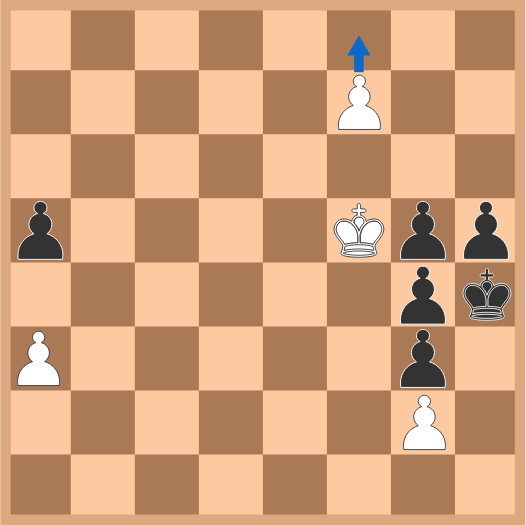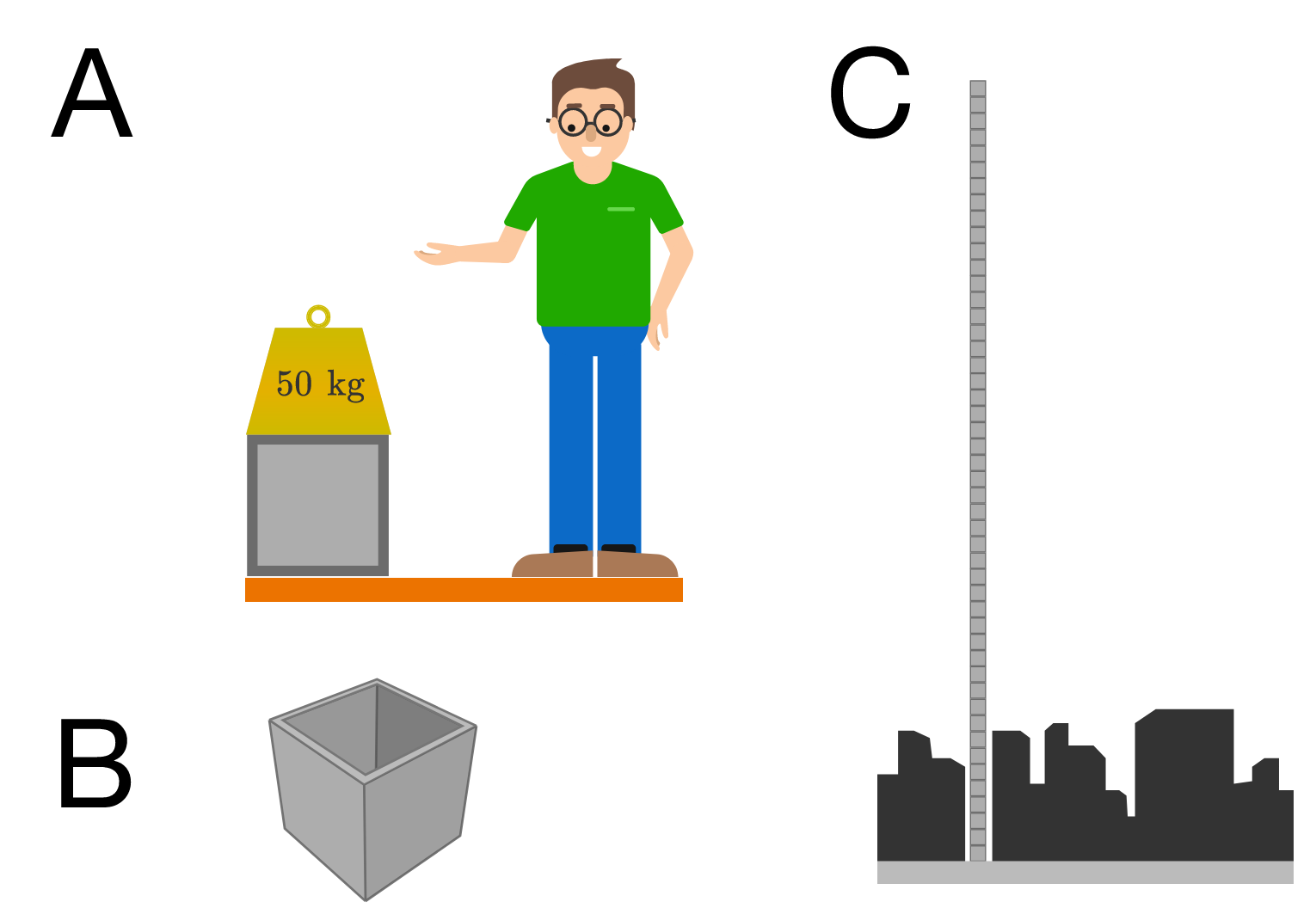# Problems of the Week

Contribute a problem

# 2018-05-07 Intermediate

White is about to advance a pawn to the last row and promote it. If White picks the wrong promotion, Black can force a draw by stalemate.

Which piece should White promote the pawn to?$1, 2, 3, 4, \ldots , 15, 16, 17$ The first $17$ positive integers are rearranged into a sequence such that the sum of any two adjacent terms is a perfect square.

What is the sum of the first and last terms of this sequence?

Integers $x$ and $y$ are such that $xy+1$ is divisible by 24.

Is $x+y$ also divisible by 24?

A unit circle is divided into 12 congruent regions, as shown. What is the perimeter of one of these regions?You're visiting a burgeoning architectural firm that wants to design a skyscraper that stands strong and tall above the local city skyline. One of the architects storms into the lunchroom with a grand announcement: they've built an incredible model for a floor of the building. It weighs just $\approx\SI{0.1}{\kilo\gram}$ yet it can sustain a $W = \SI{50}{\kilo\gram}$ weight before it breaks. Extrapolating from the test, they figure that a full-scale model built using the same material could reach 500 stories!A: the scale model, B: layout of the unit floor, C: the human-scale building.

This sounds suspicious, so you decide to do some quick figuring to estimate how tall their design could possibly stand under its own weight. Approximately how many stories would a human-scale version of a building with this floor plan sustain?

Details:

• Each floor has the dimensions of a cube of side length $\ell$, and a fraction $f = 0.7$ of the volume is missing to make room for occupants.
• The architect's model floor has linear dimension $\ell_0 = \SI{10}{\centi\meter},$ and the material has a density of $\rho = \SI[per-mode=symbol]{340}{\kilo\gram\per\meter\cubed}.$
• The height of one story in a human-scale building is $\SI{3}{\meter}.$
×